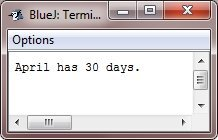# Java One Dimensional Array

A one dimensional array is, basically, a list of like-typed variables.

## Create One Dimensional Array

To create an array, you first must create an array variable of the desired data type. Here is the general form of a one-dimensional (1D) array declaration :

`type var-name[];`

Here, type declares the element type (called the base type) of the array. The element type determines the data type of each element that comprises the array. Therefore, the element type for the array determines what type of data the array will hold. For instance, below code fragment declares an array named month_days with the type "array of int" :

`int month_days[];`

Although this declaration establishes the fact that the month_days is an array variable, no array actually exists. To link month_days with an actual, physical array of integers, then you must allocate one using the new operator/keyword and assign it to month_days. The new is a special operator that allocates memory.

You will learn more about the new operator in later chapter, but you have to use it now to allocate memory for arrays. The general form of new as it applies to one-dimensional (1D) arrays appears as follows :

`array-var = new type[size];`

Here, type specifies the type of data being allocated, size specifies the number of elements present in the array, and array-var is the array variable that is linked to the array. That is, to use new to allocate an array, you must define the type and the number of elements to allocate.

The elements in the array allocated by the new will automatically be initialized to zero (for the numeric types), false (for boolean), or null (for the reference type, described in a later chapter). This example allocates a 12-element array of integers and links them to month_days:

`month_days = new int;`

When this statement executes, month_days will refer to an array of 12 integers. Further, all the elements in the array will be initialized to zero.

## Get an Array

Getting an array is a two-step process. First, you must declare a variable of the desired array type. Secondly, you must allocate the memory that will hold the array, using the new operator, and assign it to the array variable.

When you have allocated an array, you can access a specific element in the array by specifying its index within square brackets. All the arrays indexes starts from zero. For example, the following statement allots the value 28 to the second element of month_days:

`month_days = 28;`

The next line displays the value stored at index 2:

`System.out.println(month_days);`

## Java One Dimensional Array Example

Putting together all the pieces of code, below is a program that creates an array of the number of days in each month:

```/* Java Program Example - Java One-Dimensional (1D) Array */

public class JavaProgram
{
public static void main(String args[])
{

int month_days[];
month_days = new int;

month_days = 31;
month_days = 28;
month_days = 31;
month_days = 30;
month_days = 31;
month_days = 30;
month_days = 31;
month_days = 31;
month_days = 30;
month_days = 31;
month_days = 30;
month_days = 31;

System.out.println("April has " + month_days + " days.");

}
}```

When the above Java program is compile and executed, it will produce the following output:Java array indexes start from zero, so the number of days in April is month_days or 30.

It is possible to combine the declaration of array variable with the allocation of the array itself, shown below

`int month_days[] = new int;`

This is the way that you will generally see it done in professionally written Java programs.

An Array can be initialized when they are declared. The process is much the same as, used to initialize the simple types. An array initializer is a list of comma-separated expressions surrounded through curly braces. The commas separate the values of array elements. The array will automatically created large enough to hold the number of elements that you define in the array initializer. There is no need to use the new operator. For instance, to store the number of days in each month, here this code creates an initialized array of integers :

```/* Java Program Example - Java One-Dimensional Array */
/* This is the improved version of the previous program */

public class JavaProgram
{
public static void main(String args[])
{

int month_days[] = { 31, 28, 31, 30, 31, 30, 31, 31, 30, 31, 30, 31 };
System.out.println("April has " + month_days + " days.");

}
}```

The output of the above Java program is same as of the previous Java program, i.e.:## Examples

Here are some example programs, uses one dimensional array, that you can go for:

Java Online Test

« Previous Tutorial Next Tutorial »